# 基于目标检测的海上舰船图像超分辨率研究Research on Super-Resolution of Marine Ship Image Based on Target Detection

DOI: 10.12677/JISP.2019.83017, PDF, HTML, XML, 下载: 467  浏览: 1,157

Abstract: Aiming at the problem that the effective pixels in the image of marine ships account for a small proportion in the total pixels, a super-resolution method based on target detection network is proposed. The method consists of two stages, combining with the bicubic transform, to restore the sharpness of the image from coarse to fine step by step. Firstly, in the first stage, super-resolution regions in the original image are detected through the target detection network. Then, in the se-cond stage, the corresponding regions are adjusted to the specified resolution by bicubic trans-formation, and then the image details are enhanced by generating the countermeasure network. Finally, the experimental results on the self-built dataset show that compared with the traditional method and the existing super-resolution reconstruction algorithm based on deep neural network, this algorithm not only has the best visual effect, but also improves the peak signal-to-noise ratio (PSNR) of the dataset by an average of 0.79 dB and the structural similarity (SSIM) by an average of 0.04, which proves the effectiveness of the algorithm.

1. 引言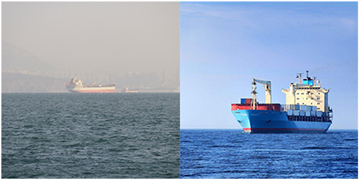Figure 1. Marine ship image

2. 研究背景及现状

2.1. 目标检测算法

2.2. 超分辨率算法

3. 基于目标检测网络的超分辨率重建模型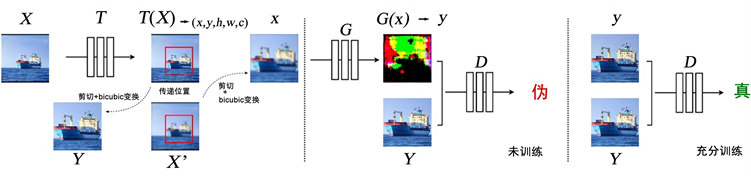Figure 2. Model structure

X为原图， ${X}^{\prime }$ 为X的退化图像，Y为X中用户感兴趣的区域，x为  中用户感兴趣的区域，y为生成网络生成的图像。T为目标检测网络，用于获取X中的(x, y, h, w, confidence)信息，G为图像生成网络，D为鉴别网络。

3.1. 目标检测卷积神经网络结构设计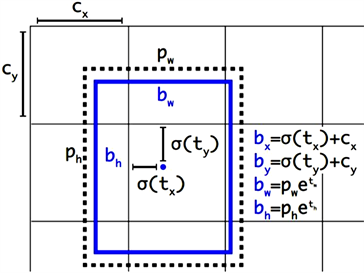Figure 3. Anchor Candidate Box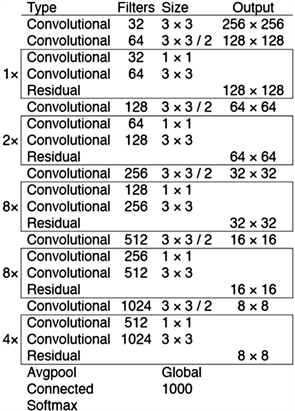Figure 4. Convolutional neural network architecture

$\begin{array}{c}loss={\lambda }_{coord}\underset{i=0}{\overset{l.w\ast l.h}{\sum }}\underset{j=0}{\overset{l.n}{\sum }}{1}_{ij}^{obj}\left[{\left({x}_{i}-{\stackrel{^}{x}}_{i}\right)}^{2}+{\left({y}_{i}-{\stackrel{^}{y}}_{i}\right)}^{2}\right]\\ \text{\hspace{0.17em}}\text{\hspace{0.17em}}+{\lambda }_{coord}\underset{i=0}{\overset{l.w\ast l.h}{\sum }}\underset{j=0}{\overset{l.n}{\sum }}{1}_{ij}^{obj}\left[{\left(\sqrt{{w}_{i}}-\sqrt{{\stackrel{^}{w}}_{i}}\right)}^{2}+{\left(\sqrt{{h}_{i}}-\sqrt{{\stackrel{^}{h}}_{i}}\right)}^{2}\right]\\ \text{\hspace{0.17em}}\text{\hspace{0.17em}}+{\lambda }_{noobj}\underset{i=0}{\overset{l.h\ast l.w}{\sum }}\underset{j=0}{\overset{l.n}{\sum }}{1}_{ij}^{noobj}{\left({C}_{i}-{\stackrel{^}{C}}_{i}\right)}^{2}+{\lambda }_{obj}\underset{i=0}{\overset{l.h\ast l.w}{\sum }}\underset{j=0}{\overset{l.n}{\sum }}{1}_{ij}^{obj}{\left({C}_{i}-{\stackrel{^}{C}}_{i}\right)}^{2}\end{array}$

3.2. 图像生成卷积神经网络结构设计

3.3. 图像判别卷积神经网络结构设计

3.4. 超分辨网络的目标函数

${\mathcal{L}}_{cGAN}\left(G,D\right)={\mathbb{E}}_{x,y}\left[\mathrm{log}D\left(x,y\right)\right]+{\mathbb{E}}_{x,z}\left[\mathrm{log}\left(1-D\left(x,G\left(x,z\right)\right)\right)\right]$

${\mathcal{L}}_{L1}\left(G\right)={\mathbb{E}}_{x,y,z}\left[{‖y-G\left(x,z\right)‖}_{1}\right]$

$G=\mathrm{arg}\underset{G}{\mathrm{min}}\underset{D}{\mathrm{max}}{\mathcal{L}}_{cGAN}\left(G,D\right)+\lambda {\mathcal{L}}_{L1}\left(G\right)$

4. 实验结果与分析Table 1. Test results on datasets using different super-resolution methodsFigure 5. Comparison of results of different super-resolution methods at 4-fold scaling ratio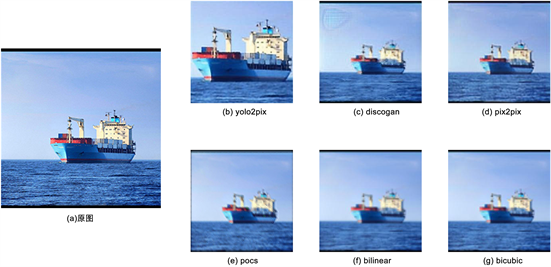Figure 6. Comparison of results of different super-resolution methods at 9-fold scaling ratio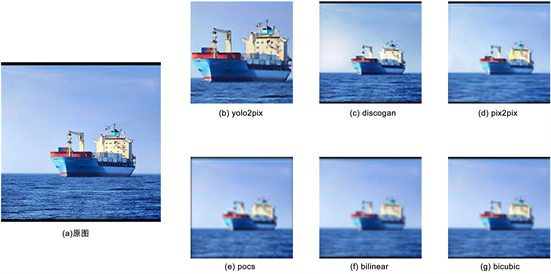Figure 7. Comparison of results of different super-resolution methods at 16-fold scaling ratio

5. 结语

  李欣, 崔子冠, 朱秀昌. 超分辨率重建算法综述[J]. 电视技术, 2016, 40(9): 1-9.  Glasner, D., Bagon, S. and Irani, M. (2009) Super-Resolution from a Single Image. In: Proceedings of the 2009 IEEE 12th International Conference on Computer Vision, IEEE, Piscataway, 349-356. https://doi.org/10.1109/ICCV.2009.5459271  郑伟成. 基于深度学习的目标检测算法综述[C]//中国计算机用户协会网络应用分会. 中国计算机用户协会网络应用分会2018年第二十二届网络新技术与应用年会论文集. 北京: 中国计算机用户协会网络应用分会, 北京联合大学北京市信息服务工程重点实验室, 2018: 5.  Redmon, J., Divvala, S., Girshick, R., et al. (2015) You Only Look Once: Unified, Real-Time Object Detection. Proceedings of CVPR 2015, Boston, 7-12 June 2015, 779-788. https://doi.org/10.1109/CVPR.2016.91  Zhong, Z., Jin, L. and Xie, Z. (2015) High Performance off Line Handwritten Chinese Character Recognition Using Google Net and Directional Feature Maps. International Conference on Document Analysis and Recognition, Nancy, 23-26 August 2015, 846-850. https://doi.org/10.1109/ICDAR.2015.7333881  南方哲, 钱育蓉, 行艳妮, 赵京霞. 基于深度学习的单图像超分辨率重建研究综述[J/OL]. 计算机应用研究, 1-7. http://kns.cnki.net/kcms/detail/51.1196.TP.20181219.1953.003.html, 2019-04-03.  Dong, C., Loy, C.C., He, K., et al. (2014) Learning a Deep Convolutional Network for Image Super-Resolution. In: Computer Vision ECCV 2014, Springer International Pub-lishing, Berlin, 184-199. https://doi.org/10.1007/978-3-319-10593-2_13  Ledig, C., Theis, L., Huszar, F., et al. (2016) Photo-Realistic Single Image Super-Resolution Using a Generative Adversarial Network. Conference on Computer Vision and Pattern Recognition, Honolulu, 21-26 July 2017, 105-114. https://doi.org/10.1109/CVPR.2017.19  Goodfellow, I., Pouget-Abadie, J., Mirza, M., Xu, B., Warde-Farley, D., Ozair, S., Courville, A. and Bengio, Y. (2014) Generative Adversarial Nets. In: Advances in Neural Information Processing Systems, The MIT Press, Cambridge, 2672-2680.  Isola, P., Zhu, J.Y., Zhou, T., et al. (2016) Image-to-Image Translation with Conditional Adver-sarial Networks. Conference on Computer Vision and Pattern Recognition, Honolulu, 21-26 July 2017, 5967-5976. https://doi.org/10.1109/CVPR.2017.632  Ronneberger, O., Fischer, P. and Brox, T. (2015) U-Net: Convolutional Networks for Biomedical Image Segmentation.  Li, C. and Wand, M. (2016) Pre-Computed Real-Time Texture Synthesis with Markovian Generative Adversarial Networks. European Conference on Computer Vision, Amsterdam, 8-16 October 2016, 702-716. https://doi.org/10.1007/978-3-319-46487-9_43  Keys, R. (2003) Cubic Convolution Interpolation for Digital Image Pro-cessing. IEEE Transactions on Acoustics, Speech, and Signal Processing, 29, 1153-1160. https://doi.org/10.1109/TASSP.1981.1163711  Kim, T. (2017) Learning to Discover Cross-Domain Relations with Gener-ative Adversarial Networks.  杨庆怡, 黄灿, 张琼. 基于POCS算法的超分辨率图像重建技术[J]. 计算机光盘软件与应用, 2012(16): 61-62.